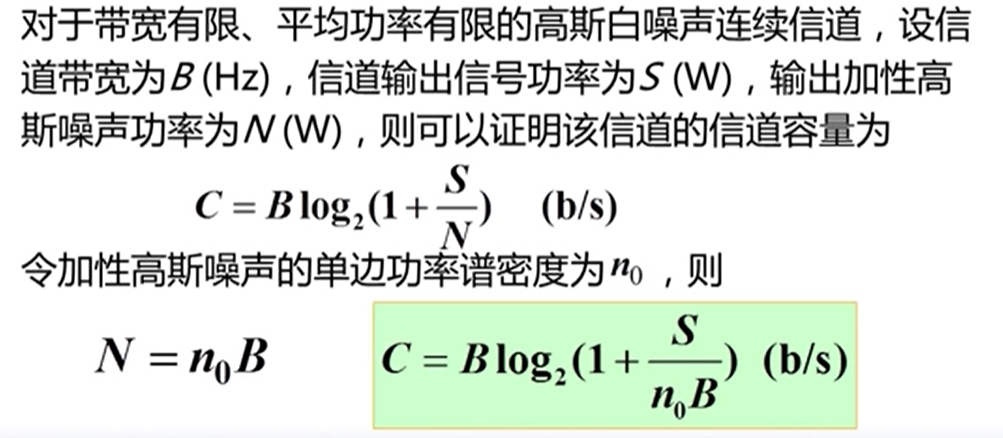• 一.香农公式 带宽极限时的信道容量
一.香农公式带宽极限时的信道容量：展开全文• MIMO信道分析，MIMO信道容量计算，对分集和复用原理介绍，以及其信道容量的计算
• 主要基于小论文提出的公式，计算了OFDM-MIMO系统的信道容量(N=64，即子载波数等于64)，当N=1时，OFDM-MIMO系统即退化为MIMO系统。通过对比发现，OFDM-MIMO系统的信道容量比单纯MIMO系统的信道容量大，感兴趣的朋友...
• 在不同的《信息论》教材中，有关信道对称性的描述并不统一，这为学习对称性信道的信道容量计算方法造成的一定障碍。因此，本文在文章的开篇部分将对本文中所描述的三种具有对称性的信道进行严格定义，以减小文章出现...
1. 三类具有对称性的信道
在不同的《信息论》教材中，有关信道对称性的描述并不统一，这为学习对称性信道的信道容量计算方法造成的一定障碍。因此，本文在文章的开篇部分将对本文中所描述的三种具有对称性的信道进行严格定义，以减小文章出现歧义的概率。

Symmetric Channel：
对称信道（Symmetric Channel）是最严格的对称性信道，该信道要求：

对于任意输入符号，其转移概率均为第一个输入符号转移概率的置换；即：
$\forall x_t\in X, \quad\exist \mathbf{E} = \prod \mathbf{E_{ij}},\quad s.t.\;\vec{p}(y|x_t) = \vec{p}(y|x_1)\mathbf{E}$
其中$\mathbf{E_{ij}}$是初等置换矩阵，$\vec{p}(y|x_t)$是第$t$个输入符号对应的转移概率向量，该向量是转移概率矩阵中的第$t$行。
对于任意输出符号，其转移概率均为第一个输出符号转移概率的置换；即：
$\forall y_t\in Y, \quad\exist \mathbf{E} = \prod \mathbf{E_{ij}},\quad s.t.\;\vec{p}(y_t|x) = \mathbf{E}\vec{p}(y_1|x)$
其中$\vec{p}(y_t|x)$是第$t$个输出符号对应的转移概率向量，该向量是转移概率矩阵中的第$t$列。

从信道矩阵观察，对称信道的任意一行的元素都完全相同，只是顺序发生了改变；任意一列也有相同的性质。

Wealky Symmetric Channel：
弱对称信道（Weakly Symmetric Channel）是对称信道的扩展，对称信道是弱对称信道的特例。相比对称信道，弱对称信道的条件约束更少，但性质却与对称信道基本相同。该信道要求：

对于任意输入符号，其转移概率均为第一个输入符号转移概率的置换；即：
$\forall x_t\in X, \quad\exist \mathbf{E} = \prod \mathbf{E_{ij}},\quad s.t.\;\vec{p}(y|x_t) = \vec{p}(y|x_1)\mathbf{E}$
其中$\mathbf{E_{ij}}$是初等置换矩阵，$\vec{p}(y|x_t)$是第$t$个输入符号对应的转移概率向量，该向量是转移概率矩阵中的第$t$行。
对于任意输出符号，其转移概率之和为常数；即：
$\forall y_t \in Y, \quad \sum_{x_i\in X}p(y_t|x_i) = C$

Quasi Symmetric Channel:
准对称信道（半对称信道，Quasi Symmetric Channel）是约束最弱的对称信道，对称信道与弱对称信道均是准对称信道的特例。该信道要求：

存在一种分块方式，$s.t.\;\mathbf{P} =[\mathbf{p_1},\mathbf{p_2},\cdots]$，且$\mathbf{p_1},\mathbf{p_2},\cdots$分块矩阵均满足对称信道条件。

下面列举的三个信道矩阵中，$\mathbf{P_1}$是对称信道，自然也属于弱对称信道和准对称信道；$\mathbf{P_2}$是弱对称信道，但也属于准对称信道；$\mathbf{P_3}$只是准对称信道。
$\mathbf{P_1} = \begin{bmatrix} 0.3 & 0.7\\ 0.7 & 0.3 \end{bmatrix}\quad \mathbf{P_2} = \begin{bmatrix} 0.4 & 0.1 & 0.2 & 0.3\\ 0.1 & 0.4 & 0.3 & 0.2 \end{bmatrix}\quad \mathbf{P_3}= \begin{bmatrix} 0.7 & 0.2 & 0.1\\ 0.2 & 0.7 & 0.1 \end{bmatrix}$
2. 对称性信道的信道容量计算
2.1. 对称信道
任何信道的平均互信息都可以写成信宿熵与噪声熵的差值，即：
$I(X;Y) = H(Y) - H(Y|X)$
噪声熵是一种条件熵，是条件事件$(y|x)$所对应的概率空间包含的信息量的期望，即：
$H(Y|X) = -\sum_{x_i\in X, y_j\in Y} p(x_i,y_j)\log_2p(y_j|x_i)$
考虑到期望运算的加权因子，即联合概率$p(x,y)$，实际上取决于信源概率分布和信道转移概率，即：
$p(x_i,y_j) = p(x_i) p(y_j|x_i)$
可以把噪声熵改写成另一种形式，在该形式中，信源分布和信道转移概率对噪声熵的影响被分离：
\begin{aligned} H(Y|X) &= -\sum_{x_i\in X, y_j\in Y} p(x_i,y_j)\log_2p(y_j|x_i)\\ &= -\sum_{x_i\in X}\sum_{y_j\in Y}p(x_i)p(y_j|x_i)\log_2p(y_j|x_i)\\ &= \sum_{x_i\in X}p(x_i)\left[ -\sum_{y_j\in Y} p(y_j|x_i)\log_2p(y_j|x_i) \right] \end{aligned}
考虑到对称信道的性质：对于任何输入符号，其转移概率分布都是第一个符号分布的置换，因此：
$\forall x_i\in X, \quad -\sum_{y_j\in Y} p(y_j|x_i)\log_2p(y_j|x_i) = C$
于是，信源分布不再对噪声熵有任何影响。噪声熵仅与信道转移概率有关，且转移概率矩阵的任意一行均可用来求解噪声熵：
\begin{aligned} H(Y|X) &= \sum_{x_i\in X}p(x_i)\left[ -\sum_{y_j\in Y} p(y_j|x_i)\log_2p(y_j|x_i) \right]\\ &= C\sum_{x_i\in X}p(x_i)\\ &= C\\ &= -\sum_{y_j\in Y} p(y_j|x_t)\log_2p(y_j|x_t) \quad \forall x_t \in X \\ \end{aligned}
于是，求解$I(X;Y) = H(Y) - H(Y|X)$在$\vec{p}(x)$上最大值的问题，转变为求解$H(Y)$在$\vec{p}(x)$最大值的问题。
信宿熵的本质也是一个概率空间的自信息熵。根据自信息熵的性质，当且仅当概率空间服从均匀分布时，自信息熵取得最大值。因此，可以试图让信宿分布达到均匀分布，进而使平均互信息最大。
需要注意的是，这样的尝试可能是失败的，因为信宿分布并不是一个可以调整的自变量。信宿分布是信源分布和信道转移概率作用后的结果，在给定信道的条件下，尝试让信宿服从均匀分布的过程就是在寻找一个合适的信源分布的过程。对于任意信道，这种信源分布很可能是不存在的（在后文求解准对称信道的章节中可以看到这一点）；但值得庆幸的是，对于对称信道，这种信源分布一定存在：
$\forall y_t\in Y,\quad p(y_t) = \sum_{x_i\in X}p(x_i,y_t) = \sum_{x_i\in X}p(x_i)p(y_t|x_i)$
需要注意的是，对称信道任意输出的转移概率分布都相同。即，只要$p(x_i)$与$x_i$无关，$\sum_{x_i}p(y_t|x_i)$就与$y_t$无关：
$p(y_t) = p(x_i)\sum_{x_i\in X}p(y_t|x_i)$
即：对于对称信道，当输入为均匀分布时，输出也是均匀分布。
综上，可以得出结论：对称信道的在输入为均匀分布时可以达到信道容量，信道容量的表达式为：
$C = \log_2r - H(Y|x_t)$
其中$r$是输出符号的数量，$H(Y|x_t)$是以任意一个输入符号为条件，输出符号空间的条件熵。
2.2. 弱对称信道
由于弱对称信道和对称信道均要求信道转移概率关于不同输入对称，因此，弱对称信道的噪声熵也与输入分布无关：
$H(Y|X)= -\sum_{y_j\in Y} p(y_j|x_t)\log_2p(y_j|x_t) \quad \forall x_t \in X$
因此，弱对称信道的信道容量也在信宿熵最大时取得。考虑到（2.1.）中求解信宿服从均匀分布时，只要求以下方程成立：
$p(y_t) = p(x_i)\sum_{x_i\in X}p(y_t|x_i)$
而弱对称信道的转移概率虽然关于不同输出并不对称，但其概率之和是定值，即$\sum_{x_i}p(y_t|x_i) = C$。因此，弱对称信道也能在均匀分布的信源作用下得到均匀分布的信宿。
因此，弱对称信道的信道容量与对称信道完全相同：弱对称信道在输入为均匀分布时可以达到信道容量，信道容量的表达式为：
$C = \log_2r - H(Y|x_t)$
其中$r$是输出符号的数量，$H(Y|x_t)$是以任意一个输入符号为条件，输出符号空间的条件熵。
2.3. 准对称信道
准对称信道的信道转移概率关于输入符号也是对称的，因此也具有对称信道和弱对称信道的关于噪声熵的结论：
$H(Y|X)= -\sum_{y_j\in Y} p(y_j|x_t)\log_2p(y_j|x_t) \quad \forall x_t \in X$
那么，求解信道平均互信息的最大值，理应也转化成求解信宿熵的最大值。与之前一样，我们期望存在一种信源分布，能使得信宿熵能实现均匀分布。然而令人遗憾的是，这种信源分布未必存在。
我们假设存在这样一种信源分布，能使信宿分布概率均为$1/r$，即：
$\begin{bmatrix} p(y_1|x_1) & p(y_2|x_1) & \cdots & p(y_r|x_1)\\ p(y_1|x_2) & p(y_2|x_2) & \cdots & p(y_r|x_2)\\ \vdots & \vdots & \ddots & \vdots\\ p(y_1|x_s) & p(y_2|x_s) & \cdots & p(y_r|x_s) \end{bmatrix}^T\times \begin{bmatrix} p(x_1)\\ p(x_2)\\ \vdots\\ p(x_s) \end{bmatrix}= \begin{bmatrix} 1/r\\ 1/r\\ \vdots\\ 1/r \end{bmatrix}$
能使输出达到均匀分布的信源分布是该线性非齐次方程组的一组解，但为了保证这个解确实能构成合理的信源概率空间，还需要添加概率空间完备性的约束条件$\sum_i p(x_i) = 1$，于是方程变为：
$\begin{bmatrix} p(y_1|x_1) & p(y_1|x_2) & \cdots & p(y_1|x_s)\\ p(y_2|x_1) & p(y_2|x_2) & \cdots & p(y_2|x_s)\\ \vdots & \vdots & \ddots & \vdots\\ p(y_r|x_1) & p(y_r|x_2) & \cdots & p(y_r|x_s)\\ 1& 1& \cdots & 1 \end{bmatrix}\times \begin{bmatrix} p(x_1)\\ p(x_2)\\ \vdots\\ p(x_s) \end{bmatrix}= \begin{bmatrix} 1/r\\ 1/r\\ \vdots\\ 1/r\\ 1 \end{bmatrix}$
该线性非其次方程组有解的充要条件是：系数矩阵与增广矩阵同秩。
未完。。。


展开全文• 论文编号:XXLW084论文字数:11049,...而信道容量计算是一个比较复杂的问题，所以我们要借助于数学软件Matlab来解决这个难题。本文的第一部分从信道容量的基本概念、基本原理、信道模型及分类等方面系统的介绍了信...
论文编号:XXLW084 论文字数:11049,页数:32摘  要信道是信息传递的通道，承担信息的传输和储存的任务，是构成通信系统的重要组成部分。信道容量是指信道能够传输信息量的大小。信道容量的研究在现实中有着非常重要的理论意义。而信道容量的计算是一个比较复杂的问题，所以我们要借助于数学软件Matlab来解决这个难题。本文的第一部分从信道容量的基本概念、基本原理、信道模型及分类等方面系统的介绍了信道容量。并在此基础上，介绍了一般信道容量的计算步骤。本文的第二部分开始介绍信道容量的迭代算法及迭代算法在Matlab中的实现，举例检验迭代算法在Matlab中实现的程序的可行性，分析程序，并与拉格朗日乘子法进行比较。关键词：信道容量  Matlab  迭代算法AbstractChannel is a channel of information transmission. And it take on the task of information transmission and storage. Channel is an important part of communication system. Channel capacity is the size of the amount of information can be transmitted. It has important significances in reality. However, calculating the channel capacity is a complex issue. So we must use the mathematical software Matlab to solve this problem.The first part of the article, it introduces channel capacity by the basic concepts, principles and the classification of channel models. On this basis, introduce and discuss the calculation steps of the general channel capacity.The second part of the article, it introduces the Iterative algorithm of the channel capacity and implementes the iterative algorithm in Matlab. After that, by realizing the feasibility of the procedure, we make some examples. And also analyze the procedure. Then compare it with Lagrange multiplier method.Keywords: channel capacity   Matlab    Iterative algorithm目 录中文摘要  i英文摘要  ii目 录 iii第一章  前言 11.1  论文研究的背景 11.2  研究信道容量的目的 11.3  研究信道容量的意义 2第二章  离散无记忆信道及信道容量 32.1  信道 32.2  信道模型及其分类 32.2.1  信道模型 52.2.2  信道分类 52.3  离散信道的数学模型 62.4  离散无记忆信道 72.5  信道容量的基本概念 7第三章  离散信道容量的计算 83.1  特殊离散信道的信道容量计算 83.1.1  离散无噪信道的信道容量 93.1.2  离散对称信道的信道容量 103.2  一般离散信道的信道容量计算 15第四章  信道容量的迭代算法及其实现 164.1  迭代算法 194.2  迭代算法在Matlab中的实现 204.3  信道容量的算例及其在Matlab中的实现 234.4  程序分析 234.5  拉格朗日乘子法与迭代算法的比较 25第五章  总结 26致  谢 27参考文献 28本站会员可自行下载:如何获取积分?基于Matlab的信道容量计算相关论文
展开全文• 陕西科技大学 信息论实验报告 信息论实验 信道容量计算 霍夫曼编码 算术编码
• TD-SCDMA单载频小区信道容量计算（一） TD-SCDMA单载频小区信道容量计算   作者：苏华鸿 梁天恩 熊金州  0 前言  TD-SCDMA作为TDD模式技术，比FDD更适用于上下行不对称的业务环境，是多时隙TDMA与直扩...

这篇文章解答了我很多疑问

TD-SCDMA单载频小区信道容量计算（一）

TD-SCDMA单载频小区信道容量计算

作者：苏华鸿 梁天恩 熊金州

0 前言

TD-SCDMA作为TDD模式技术，比FDD更适用于上下行不对称的业务环境，是多时隙TDMA与直扩CDMA技术合成的新技术。

同时，TD-SCDMA标准建议所采用的空中接口技术作为当前业界最为先进的传输技术之一，通过与智能天线技术、同步CDMA等技术的融合，形成了目前频谱使用率最高、成本最低的第三代无线网络技术。

现有TD-SCDMA规范主要是针对1个小区对应1个单载频的情形，空中接口对于无线资源的操作、配置都是针对1个载频来进行的。在Iub接口小区建立的过程中1个Cell只需配置1个绝对频点号。

如果1个基站配置了多载频，则每个载频被当作1个逻辑小区，每个逻辑小区各自独立地维护1套导引信息和广播信息。因此，当1个扇区有几个载频时，其容量应是几个单载频小区容量之和。随着TD-SCDMA规范的不断完善，将会推出多载频小区，其突出优势是仅在主载频上发射导引信息，有利于减少导频信号干扰，提高系统效率。

1 TD-SCDMA物理信道

TD-SCDMA系统的物理信道采用4层结构:系统帧号、无线帧、子帧、时隙/码。依据资源分配方案的不同，子帧或时隙/码的配置结构也可能有所不同。系统使用时隙和扩频码在时域和码域上来区分不同的用户信号。

TDD模式下的物理信道由突发（Burst）构成，这些Burst仅在所分配的无线帧中的特定时隙发射。无线帧的分配可以是连续的（即每一帧的时隙都分配给物理信道），也可以是不连续的（即仅有部分无线帧中的时隙分配给物理信道）。

除下行导频（DwPTS）和上行接入（UpPTS）突发外，其他所有用于信息传输的突发都具有相同的结构，即由2个数据部分、1个训练序列码和1个保护时间片组成。数据部分对称地分布于训练序列的两端。1个突发的持续时间就定义为1个时隙。1个发射机可以在同一时刻、同一频率上发射多个突发以对应同一时隙中的不同信道，不同信道使用不同的OVSF信道化码来实现物理信道的码分。

在TD-SCDMA系统中，每个小区一般使用1个基本的训练序列码。对这个基本的训练序列码进行等长的循环移位（长度取决于同一时隙的用户数），又可以得到一系列的训练序列。同一时隙的不同用户将使用不同的训练序列位移。因此，1个物理信道是由频率、时隙、信道码、训练序列位移和无线帧分配等诸多参数来共同定义的。

1.1帧结构

3GPP定义的1个TDMA帧长度为10ms。TD-SCDMA系统为了实现快速功率控制和定时提前校准以及对一些新技术的支持（如智能天线），将1个10ms的帧分成2个结构完全相同的子帧，每个子帧的时长为5ms。每个5 ms的子帧由3个特殊时隙和7个常规时隙（TS0～TS6）组成。常规时隙用作传送用户数据或控制信息。在这7个常规时隙中，TS0总是固定地用作下行时隙来发送系统广播信息（在单载频小区，通常不承载业务），而TS1总是固定地用作上行时隙。其他的常规时隙可以根据需要灵活地配置成上行或下行，以实现不对称业务的传输，如分组数据。每个子帧总是从TS0开始。用作上行链路的时隙和用作下行链路的时隙之间由1个转换点分开。每个5
ms的子帧有2个转换点，第一个转换点固定在TS0结束处，而第二个转换点则取决于小区上、下行时隙的配置，可位于TS1～TS6结束处。

1.2时隙结构

时隙结构也就是突发的结构。TD-SCDMA系统共定义了4种时隙类型，它们是DwPTS、UpPTS、GP和TS0～TS6。其中DwPTS和UpPTS分别用作上行同步和下行同步，不承载用户数据，GP用作上行同步建立过程中的传播时延保护，TS0～TS6用于承载用户数据或控制信息。

DwPTS时隙

DwPTS时隙用来发送下行同步码（SYNC_DL），其时隙长度为96chip，其中同步码长为64chip，前面有32chip用作TS0时隙的拖尾保护。Node B必须在每个小区的DwPTS时隙发送下行同步码。不同的下行同步码标识了不同的小区，其发送功率必须保证全方向覆盖整个小区。按物理信道来划分，发送下行同步码的信道也叫做下行同步信道（DwPCH）。在DwPTS时隙没有码分复用，也就是说，该时隙仅有1个物理信道DwPCH。

UpPTS时隙

UpPTS时隙被UE用来发送上行同步码（SYNC_UL），以建立和NodeB的上行同步。UpPTS时隙长度为160chip，其中同步码长为128chip，另有32 chip用作拖尾保护。多个UE可以在同一时刻发起上行同步建立。Node B可以在同一子帧的UpPTS时隙识别多达8个不同的上行同步码。按物理信道划分，用于上行同步建立的信道也叫做上行同步信道（UpPCH）。1个小区中最多可有8个UpPCH同时存在。

TS0～TS6时隙

TS0～TS6共7个常规时隙被用作用户数据或控制信息的传输，它们具有完全相同的时隙结构。每个时隙被分成了4个域：2个数据域、1个训练系列域（Midamble）和1个用作时隙保护的空域（GP）。

1.3数据域

数据域对称地分布于Midamble码的两端，每域的长度为352chip，所能承载的数据符号数取决于所用的扩频因子。每一数据域所能容纳的数据符号数S与扩频因子SF的关系为：S×SF＝352。在TD-SCDMA系统中，上行方向SF可取的值为：1、2、4、8、16，其对应的S值为：352、176、88、44、22，而在下行方向，SF可取的值仅为1和16两种，对应的S值为352和22。

数据域用于承载来自传输信道的用户数据或高层控制信息，除此之外，在专有信道和部分公共信道上，数据域的部分数据符号还被用来承载3种类型的物理层信令：TFCI、TPC和SS，详见参考文献1。

2 TD-SCDMA单载频小区容量

2.1信道与BRU

在TD-SCDMA系统中，现有规范规定1个小区对应1个载频，1个信道就是载波、时隙、扩频码的组合，也叫1个资源单位。其中，1个时隙内由1个16位扩频码划分的信道有16个，它是最基本的资源单位，即BRU。1个信道占用的BRU个数是不一样的，1个RU（RUSF1）占用了16个BRU，1个RUSF8则占用2个BRU，通常1个语音业务信道需占用2个BRU，而在1个载波上，所能提供的BRU的最大个数是固定的。在每个RU中，即在1个常规时隙中含有2个数据符号字段，其中每个数据符号字段有352chip，则在1个RU中有352×2=704chip。当扩频因子为16时（对应1个BRU），在1个RU中所包含的数据符号数为704/16=44bit。如果采用QPSK调制方式，则在1个码道中所包含的数据比特数为44×2=88
bit；如果采用8PSK调制方式（此种调制方式一般应用于的业务），则在1个码道中所包含的数据比特数为44×3=132 bit。因为1个子帧的长度为5 ms，因此，当采用QPSK调制方式时，1个BRU的速率为88 bit/5 ms=17.6 kbit/s；当采用8PSK调制方式时，1个BRU的速率为132 bit/5 ms=26.4 kbit/s。

2.2多码道传输与单码道传输

在TD-SCDMA中，OVSF码的使用使得信道可以传输各种速率的数据：对于低速的数据可以采用较大的扩频因子（扩频增益大）；而高速的数据可以用较小的扩频因子（扩频增益小）。这样对于1个高速的（需要多个资源单元）承载业务，可以有2种信道分配方式：一是为该业务分配多个码道，其中每个码道都采用较大的扩频因子（较低的单信道数据速率），进行多码道传输，以达到较高的数据速率（如分配2个SF=16的码道）；二是仅为该业务分配1个（或者较少）码道，并使用较小的扩频因子（较高的单信道数据速率，如分配1个SF=8的码道）。

2.3时域集中分配与码域集中分配

对于多码道传输，也有2种不同的码道分配方式需要考虑：“码域集中分配”和“时域集中分配”，当然，也可以采用两者的结合。码域集中分配是首先将1个时隙内的多个码道集中分配给用户，如果该时隙内可用码道不够，再考虑分配其他时隙内的码道；而时域集中分配是同时将多个时隙分配给用户，但每个时隙可能分配更少的BRU给该用户。码域集中分配减少了每个时隙内的平均用户数，但由于在同一时隙可能同时需要多个码道，阻塞概率将高于时域集中分配原则。如下行128k数据业务，既可以使用每子帧1个时隙，每个时隙分配16个BRU（码域集中分配，简称方案一），也可以采用每个子帧2个时隙，每个时隙8BRU（偏向于时域集中，简称方案二）。假设系统能满码道工作，采用方案一，每个时隙平均可能有2个用户，而方案二则只可能有1个用户。同样，在系统不进行资源整合时，每个时隙有1个小业务量用户（譬如话音业务），则128k业务采用方案一的资源分配策略将被阻塞，而采用方案二则不会。同时，采用方案二，由于同一时隙支持的用户数较多，因此在空间上可以隔离，结合智能天线的波束赋形，小区内干扰较低，基于干扰的接纳控制时，方案二被阻塞的概率也降低了。从系统性能来看，时域集中分配总体上优于码域集中分配，但对RRM算法的要求和终端的设计要求也更高。因此，在覆盖受限的业务可以考虑时域集中分配，将所需的BRU分散到不同时隙，增大小区覆盖，而其他业务则主要考虑码域集中分配，降低RRM调度的复杂性。

2.4BRU需求量计算

在系统中，根据无线信道呼叫阻塞率指标和预测的话务量数据，可以直接查找ErlangB、ErlangC或PoissoN表得到所需的话音频道数。对应于TD-SCDMA系统，借助于爱尔兰呼损公式及计算表，也可以得到系统BRU的需求量，但计算相对复杂。下面通过1个实际的例子，介绍一下TD-SCDMA系统BRU需求量的计算方法。假设某城市支持的业务，同一种业务类型的不同速率有不同的业务ID，按不同业务处理。根据某种预测模型得到该区域的语音业务（业务1）为640Erl，业务2的通道速率为6400kbit/s，业务3的通道速率为10
666 kbit/s，业务4的通道速率为4 800 kbit/s；对于数据业务，在给定承载的前提下，可以将其转换成等效爱尔兰，即数据业务等效信道数=通道速率/承载速率，再查爱尔兰表，。要求的无线信道的呼叫阻塞率为2%。

根据不同业务的BRU分配方案，业务1每载扇需要2BRU，业务2和业务3每载扇需要8BRU，业务4每载扇需要16BRU。

业务量预期在3∶3时隙比例配置中，同1个载扇只能提供24个业务1的用户（3×16BRU每载扇/2BRU，即24用户每载扇），或6个业务2的用户，或6个业务3的用户，或3个业务4的用户。通过查找ErlangB表，得到在2%呼叫阻塞率时，1个小区支持的不同业务的等效爱尔兰数分别为：16.6、2.276、2.276和0.602Erl。通过简单的分析，该区域需要的载扇总数为：640/16.6+87.97/2.276+72.53/2.276+25.53/0.6=123，需要123个单载频小区。

展开全文• 目前3GPP LCR TDD标准适合于独立组网，属单载频小区系统，信道和BRU局限在1个载频内。规范进一步发展的重点将是多载频小区，网络性能将会有较大提高。TD- SCDMA多载频系统的相关规范目前已在中国通信标准化协会...职场管理
• 研究需要求 BSC信道+AWGN信道 的级联信道容量，于是查书解决。 原来一直想当然的以为，并联级联信道容量是各个独立信道的容量相加，那串联就是各个相乘呗。一看书才发现，并联是没错，可串联就不是这么简单了。书...
• 2015年11 月18日星期三 15:02:16 多用户上行模型 方案1：先解低速用户，再解高速用户 注： ...先解高速用户，在解低a
• 姓名：孟云飞学号Y080430 任意信道的信道容量C及最佳输入分布P_X源程序function [P_X,C,N]=contmax(P_YX,e)% 计算任意信道的信道容量C及最佳输入分布P_X% P_X -------输入概率分布% C----------信道容量N----------...
• c语言源代码，实现信道容量计算，实现了一般信道容量的迭代计算，以及迭代次数，采用信息论中的作业，编写方法详见《信息论基础》人民邮电出版社124夜的算法去编写。
• 一般信道容量的迭代计算，采用纯c语言编写，实现了输入信道转移矩阵随机，同时可以将一次输入的信道矩阵通过修改精确度来计算信道容量
• (信道容量知识总结信道容量是信道的一个参数，反映了信道所能传输的最大信息量，其大小与信源无关。对不同的输入概率分布，互信息一定存在最大值。我们将这个最大值定义为信道的容量。一但转移概率矩阵确定以后，...
• 信道能无错误传送的最大信息率。对于只有一个信源和一个信宿的单用户信道，它是一个数，单位是比特每秒或比特每符号。它代表每秒或每个信道符号能传送的最大信息量，或者说小于这个数的信息率必能在此信道中无错误地...
• 另一个是信道编码，克服信道中的干扰和噪声，提高了可靠性。可见信道是通信系统的重要组成部分，它的任务是实现信息的传输，在信道固定的情况下，总是希望传输的信息越多越好。本文主要研究一...
•研究论文
• 典型信道容量计算。二元对称信道，无损信道，确定信道，无损确定信道，离散对称信道。matlab
•c语言
• DMC 信道容量迭代计算的matlab 实现--通信与信息系统一、用了matlab 实现DMC 容量迭代的算法如下：1. 初始化信源分布：.0det a 10,1,0,1)(>>=?==，选置，，k r i rP k i 一般我选deta=0.000001。2. }{,)()()()...
• 用于MIMO信道容量计算的matlab代码，很好用。
• 该资料分析了任意信道容量计算 并用MATLAB进行了仿真和分析
• 用matlab软件编程，利用迭代算法计算信道容量
• 参考北京邮电大学出版的信息论基础教程编写的迭代法计算信道容量，MATLAB语言。
• 基于matlab gui 制作的数字通信系统中信道容量计算代码及图。
• 这个程序主要是计算信道容量的，所以有需要的朋友们下载吧c++
• 已知信道转移矩阵，通过使用迭代算法，来计算信道容量...